# Newton's 3 Laws of Motion Explained

• Author:
• Updated date:

Ray is a licensed engineer in the Philippines. He loves to write about mathematics and civil engineering.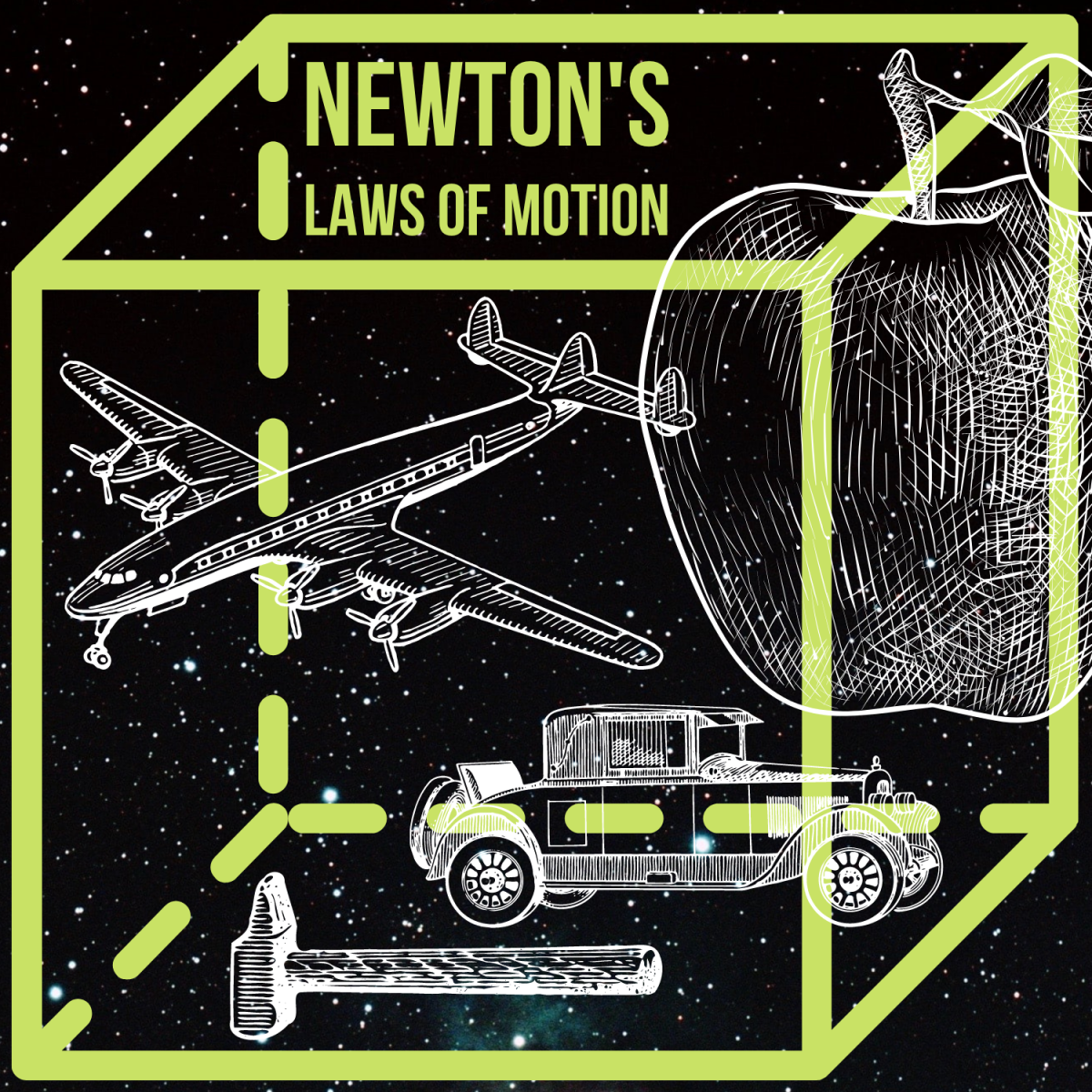Explore Newton's 3 Laws of MotionAldebaran S via Unsplash; Canva

## Who Was Isaac Newton?

In the 16th century, Galileo contributed considerably to the rapid advance of science, especially mechanics. Another great scientist, Isaac Newton (1642⁠-1727), was born the year he died, destined to continue Galileo's great work. Like Galileo, Newton was interested in experimental science, particularly that part of mechanics involving bodies in motion.

Newton was the first person to study motion fundamentally. He studied and clarified some of Galileo's ideas and proposed three laws of motion concerning relations between force and motion.

## What Are Newton's Three Laws of Motion?

1. Newton's First Law of Motion (Law of Inertia)
2. Newton's Second Law of Motion (Law of Mass and Acceleration)
3. Newton's Third Law of Motion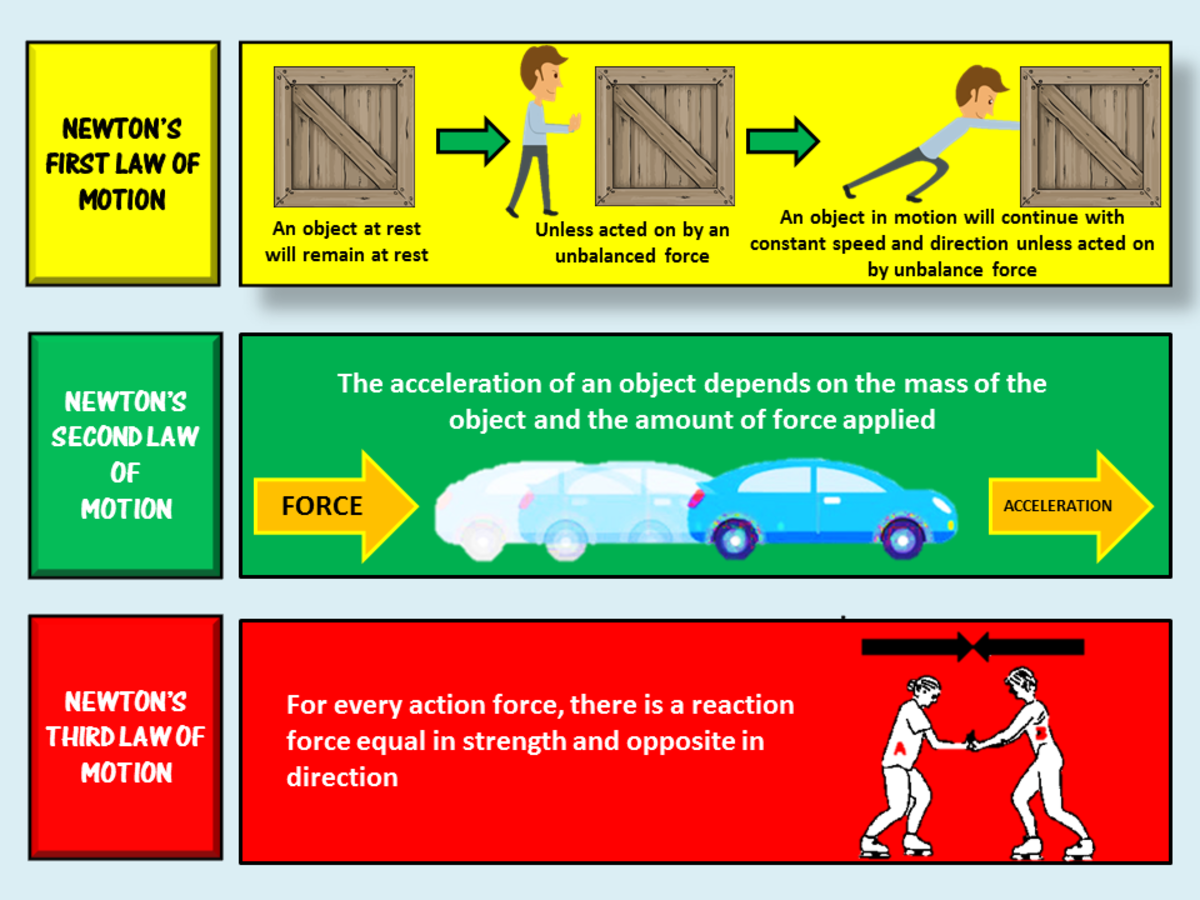The Newton's three laws of motion are Law of Inertia, Law of Mass and Acceleration, and the Third Law of Motion. John Ray Cuevas

## 1. Newton's First Law of Motion (Law of Inertia)

Galileo said that velocity is not necessarily zero if there is no force; it is acceleration which is zero if there is no force. Newton's first law of motion restated this idea, sometimes called the law of inertia.

Inertia is a property of a body that tends to preserve that body's state of rest when it is at rest or maintain its motion when it is in motion. The mass of the body is a measure of its inertia.

Example: Consider a passenger standing on a bus running at a constant speed along a straight highway. The passenger is thrown forward when the driver suddenly steps on the brakes. According to Newton's first law of motion, the passenger maintains his state of constant speed unless acted upon by an external force. To avoid being thrown forward, the passenger tries to grasp a part of the bus to hold him back.

### Two Parts of Newton's First Law of Motion

Newton's first law applies to bodies at rest and bodies in motion. Let's look at each separately.

### Body at Rest

Let us use an object lying on a table as our example. According to the first law of motion, this object will remain at rest. This state of rest can only be changed by applying an external force on the body, such that it is a net force. Two forces act upon the body as it lies on the table: These are its weight and the upward reaction exerted by the table. But these two forces alone have a zero resultant, meaning there is 0 net force on the object. The law implies that the smallest net force on the object will move it.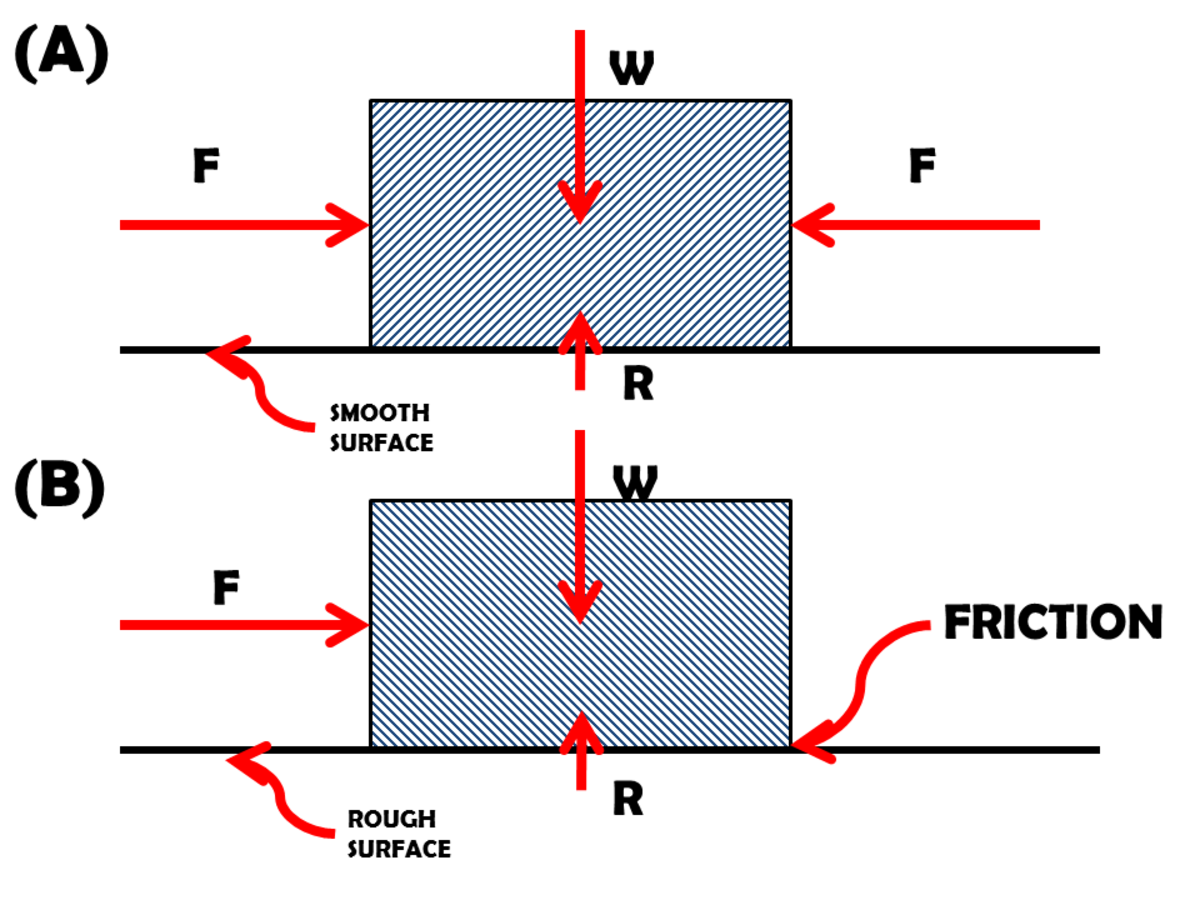Newton's First Law of Motion states that an object will remain at rest or in uniform motion in a straight line unless acted upon by an external force. John Ray Cuevas

In figure A above, the block of weight (W) is placed on a smooth surface and acted upon by two equal and opposite horizontal forces (F). The resultant of all three forces on the block is zero; hence there is no net force. According to the first law, the block will remain at rest.

In figure B above, the same block is placed on a rough surface. Its weight (W) is balanced by the upward reaction (R) of the surface. A single force (F) is applied to the block, but the block does not move. Because the surface is rough, there is a retarding force of friction directed to the left and balances the force F. Hence, all the forces form a system of forces in equilibrium. There is no net force on the block, and it will remain at rest.

Let us recall our experience standing on a bus that is at rest. Our body is also at rest. When the bus suddenly starts, we seem to be thrown backward. We are thrown backward relative to the bus, which is moving forward. Concerning the ground, however, we are trying to maintain our position at rest.

Scroll to Continue

## Read More From Owlcation

### Body in Motion

As for the second part of Newton's first law of motion, consider a body in motion. This law says that the body will remain in uniform motion along a straight line. This means that it will move at a constant speed in a fixed direction unless it is acted upon by a net external force. The state of uniform motion can change in one of the three ways listed below:

• The speed is changed, but the direction of the velocity remains constant.
• The direction of the velocity is changed, while the speed remains constant.
• Both the magnitude and the direction of the velocity are changed.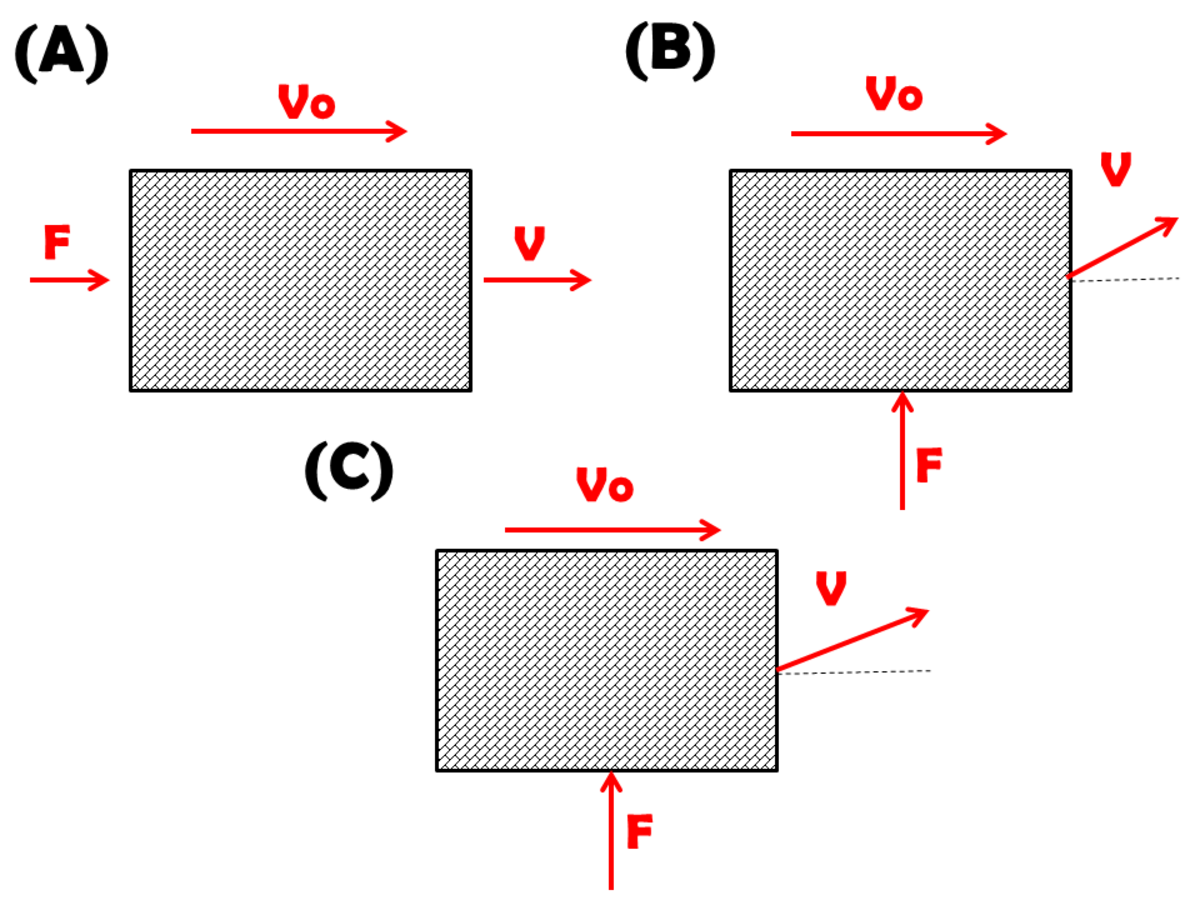Newton's first law of motion states that every object will remain at rest or in uniform motion in a straight line unless compelled to change its state by the action of an external force.John Ray Cuevas

Figure A above shows a block moving to the right with an initial velocity vo. When the force F is directed to the left of the block, the velocity is increased in magnitude, but the direction of motion is not changed. This is true whenever the force is in the same direction as the velocity.

In Figure B, the force is perpendicular to the direction of motion. Only the direction of the velocity is changed, and the magnitude remains.

In Figure C, the force is neither parallel to the direction of the velocity nor perpendicular to it. Both the magnitude and the direction of the velocity are changed.

### The Force of Friction

The force of friction is hard to remove in any object. Even an object like an airplane flying through the air encounters air resistance. This is why objects don't move continuously if no forces are acting on the body. After a body has been put into motion, it will eventually stop due to the retarding force of friction. However, following the thinking of Galileo, friction can sometimes be absent, in which case a body already moving will continue to move indefinitely at a constant speed along a straight line.

## 2. Newton's Second Law of Motion (Law of Mass and Acceleration)

The second of Newton's three laws of motion is also known as the law of mass and acceleration.

The equation F = ma is probably the most-used equation in mechanics. It states that the net force on a body is equal to the mass multiplied by the acceleration. The equation is valid provided proper units are used for the force, the mass, and the acceleration. Both sides of the equation involve vector quantities. It is implied that they must have the same direction wherein the acceleration is the same direction as the applied force. Since the acceleration is in the same direction as the change in velocity, it follows that the change in velocity due to the applied force is also in the same direction as the force.

The equation a = F/m says that the acceleration produced is proportional to the net force and inversely proportional to the mass. It can also be written as m = F/a. This equation says that the mass of a body is the ratio of the applied force to the corresponding acceleration. This is also the definition of inertial mass in terms of the two quantities that can be measured.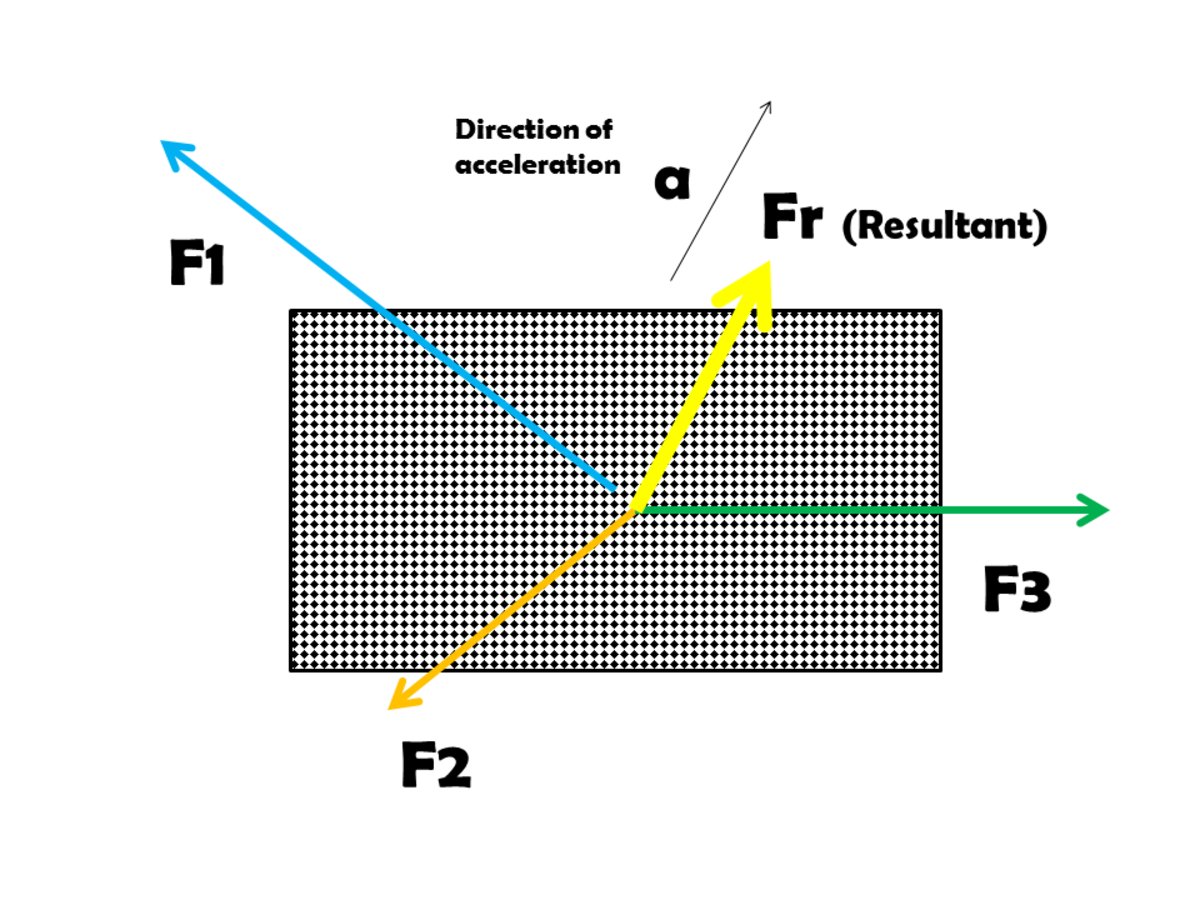Newton's second law of motion states that the acceleration of an object is dependent upon two variables: the net force acting upon the object and the mass of the object.John Ray Cuevas

### If the body is acted upon by two or more forces, what will be its acceleration?

The second law says that the acceleration is in the same direction as the net force. By "net force" we mean the resultant of all the forces acting on the body. The figure above shows a body of mass (m) acted upon by three forces. The resultant of these forces is the net force on the body, and the acceleration produced will be along the direction of this resultant.

## 3. Newton's Third Law of Motion

Newton's first two laws of motion refer to single bodies. These two laws are laws of motion. Newton's third is not a law of motion but a law of forces.

Newton's third law of motion posits that for every force applied, there is always an equal and opposite reaction. Or, if one body exerts a force on another, the second body exerts an equal and opposite force on the first. It is not possible to exert a force on a body without a reaction, and the reaction exerted by the body is exactly equal to the force being applied to the body, neither more nor less.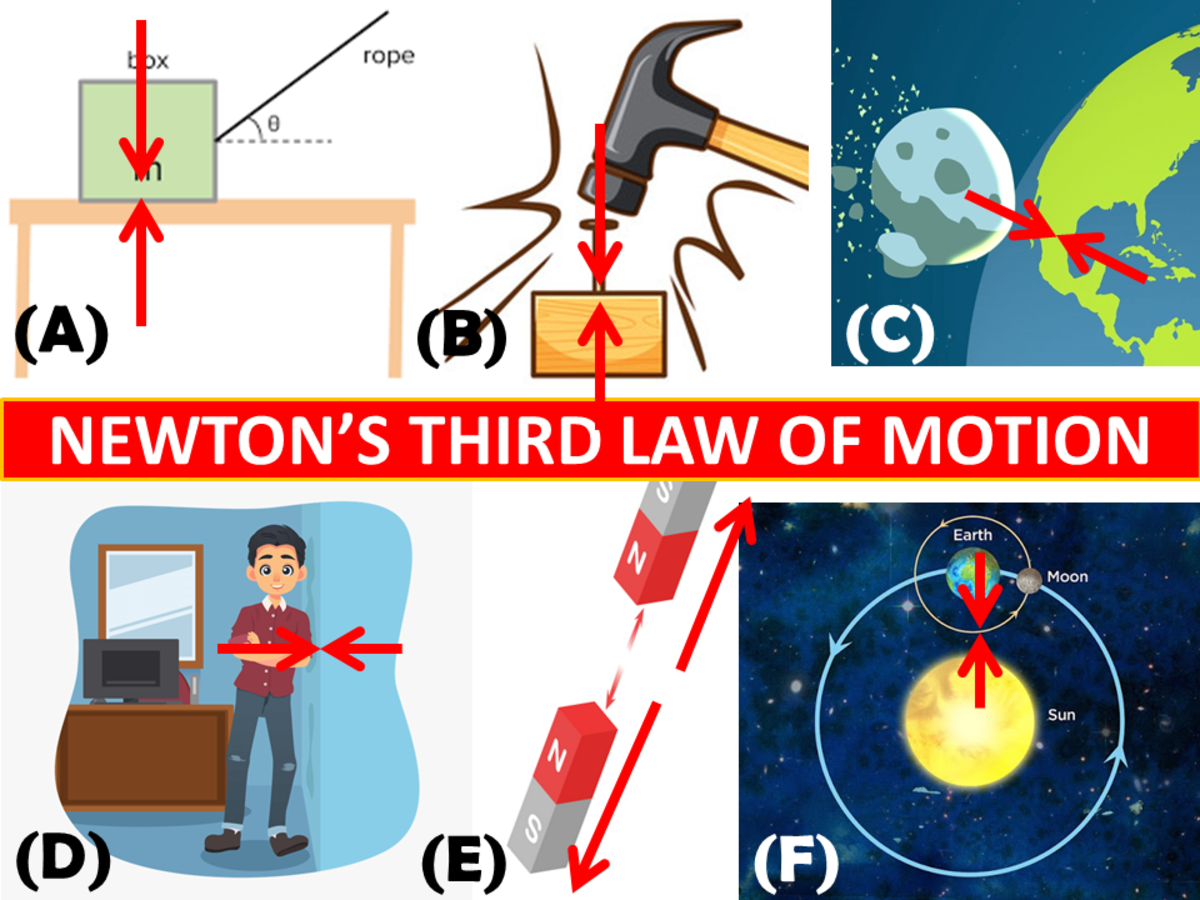Newton's third law of motion states that for every action (force) in nature there is an equal and opposite reaction.John Ray Cuevas

It must be kept in mind in all these examples that the action and reaction forces are applied to different bodies (box/table, hammer/peg, etc.).

Figure A: A block is placed on a tabletop. Two equal and opposite forces are shown, F and -F. These two forces are exerted by the block and the table on each other. What the action is and what the reaction depends on depends upon which body is being considered. If we take the tabletop as the body, then F is the action and -F the reaction. The action is the force on the body under consideration, while the reaction is the force by the body on some other body.

Figure B: A hammer is driving a peg into the ground. The two bodies are in contact only during a short interval, and both of them may be moving together. At any instant during a short interval of contact, the action and reaction are equal, even if the peg is being driven into the ground. If the hammer is taken as the body, the action is -F and the reaction by the hammer is F. On the other hand, if the peg is taken as the body, the action on it is F and the reaction by it is -F. (There is also another pair of action-reaction forces between the peg and the ground, but we are talking only the hammer-peg pair of bodies.)

Figure C: A terrestrial body is falling toward the earth's surface. When the body falls, it is being attracted by the earth, or it is being pulled by the earth. Since we cannot see the motion of the earth, the possibility of a force acting on the earth does not occur to us.

Figure D: A man is leaning against a wall. The action on the wall is force F, and the reaction by the wall is the force -F. The reaction of the wall can be only as much as the force applied to it. It seems strange that the wall is pushing the man, although we see the man pushing.

Figure E: Two magnets with their north poles are facing each other. In magnetism, like poles repel each other. The repulsive force exerted by one magnet on the other is equal and opposite to the repulsive force exerted by the second magnet on the first. This is true even if one magnet is stronger than the other.

Figure F: The third law is applied on a large scale to the sun-earth system. It was also shown by Newton that the earth is kept in its orbit around the sun by the attraction of the sun to the earth. At the same time, the earth also attracts the sun with an equal and opposite force.

## Quiz Yourself

For each question, choose the best answer. The answer key is below.

1. What is the absolute unit of force?
• Pascal
• Kilograms
• Pounds/square feet
• Newton

1. Newton

This content is accurate and true to the best of the author’s knowledge and is not meant to substitute for formal and individualized advice from a qualified professional.

Miebakagh Fiberesima from Port Harcourt, Rivers State, NIGERIA. on March 29, 2020:

Hey Ray, you're welcomed.

Ray (author) from Philippines on March 28, 2020:

Hi, Miebakagh! Yeah, I really hope I am able to turn up this topic. It basically sums up PHYSICS. Thanks for stopping by!

Ray (author) from Philippines on March 28, 2020:

Hi, Umesh! I am glad you liked my presentation of this topic. Thanks for checking out my article.

Miebakagh Fiberesima from Port Harcourt, Rivers State, NIGERIA. on March 28, 2020:

Hey Ray, although I read physics in school, here you amplified the subjects much. Thanks for sharing.

Umesh Chandra Bhatt from Kharghar, Navi Mumbai, India on March 28, 2020:

Nice article. I have also interest in Physics and write basic articles on it. Your presentation is very good.